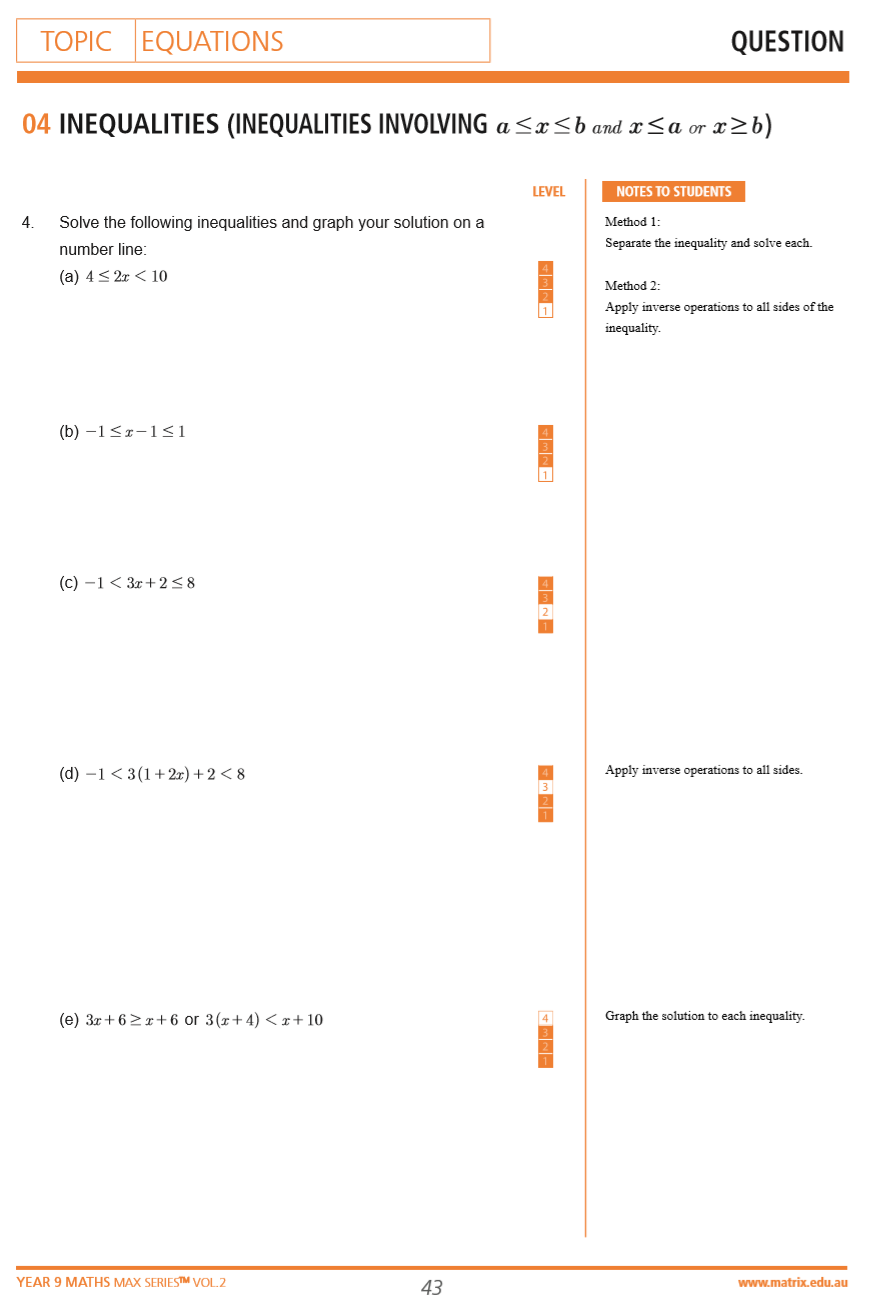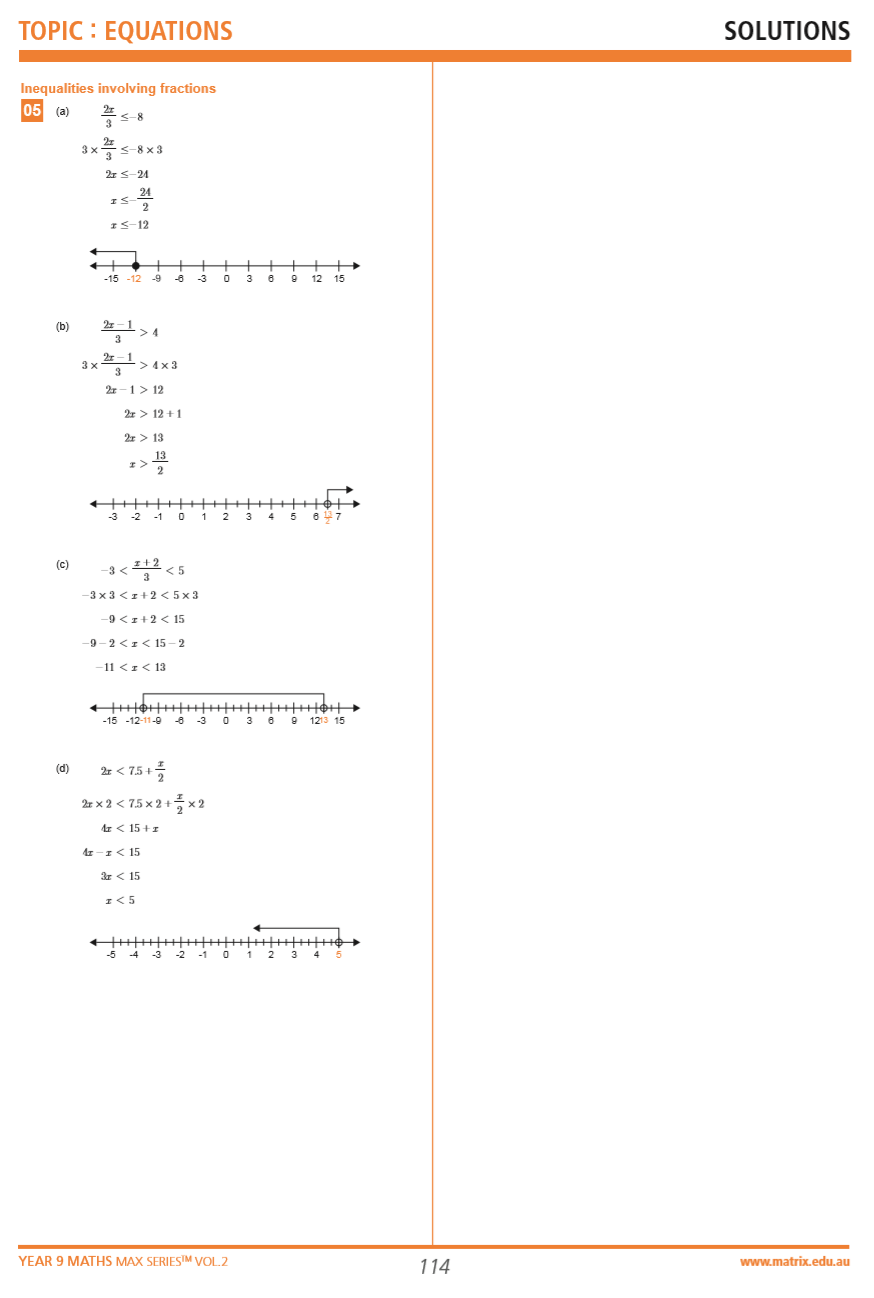# Part 2: Inequalities

In this article, we introduce you to inequalities and show you how to solve them using your algebra skills.## Inequalities

Understanding inequalities and being able to approach these type of questions is foundational knowledge that all students should know before Year 10. Have a read through this guide if you want to consolidate your abilities or even if you’re learning it for the first time!

## NSW Syllabus Outcome

This article addresses the following syllabus outcomes:

• Compare the relative value of integers, including recording the comparison by using the symbols (< and >)
• Ordering lists

In this unit, you will learn that mathematics is a concise way to write statements comparing the size of numbers.

## In this article we discuss

• How inequalities work
• Solving equations with inequalities
• Common mistakes regarding representation of inequalities
• Comparing different forms of displaying numbers (e.g. scientific notation, mixed fractions)

## Assumed knowledge

Students should be familiar with solving simple algebraic equations. They should also understand how to convert a certain number between different forms e.g. fraction to mixed fraction to decimal.

$$\frac{7}{4}=1\frac{3}{4}=1.75$$

Students must also be familiar with converting to and from index notation and scientific notation.

## What are Inequalities?

To put it simply, inequalities is a system of expressing one number as smaller or larger than another number. There are two symbols which are commonly used to represent this:

< (less than) and
(greater than)

The trick to remember this is that the two lines “opens” up to the larger number and “closes” towards the smaller number.

## Example: Fill in the blank

Fill in the blank with the appropriate inequality sign:

$$5$$ _____ $$8$$

## Solution

Since we know that 5 is less than 8, we would use the “less than” sign.

$$5<8$$

### Additionally!

There is another form of notation that will be used in senior years:

(less than or equal to)

and (greater than or equal to)

## Solving Equations with Inequalities

You need to be able to solve equations that feature inequalities. Let’s take a look at how to do that.

## Linear Equations

Normally when solving linear equations, you’d expect to find a single value of $$x$$.

### For example:

\begin{align*}
2x-1=5 \\
2x=6 \\
∴x=3 \\
\end{align*}

However, equations with an inequality sign (rather than an equals sign) means that you are solving for value(s) of $$x$$ that would satisfy the “inequality”.

$$2x-1>5$$

Here you want to find all values of x that would make $$2x-1$$ larger than $$5$$.

Fortunately, you would solve these types of equations in the same way as ordinary equations. However, there is one extra rule which will be discussed later.

i.e. Treat the inequality sign like an equals sign.

For now, consider:

\begin{align*}
2x-1>5 \\
2x>6 \\
x>3 \\
\end{align*}

By treating the inequality sign the same as an equals sign, you have answered the question. For any value of $$x$$ larger than $$3$$, our original inequality would be satisfied.
E.g if $$x=4$$, $$2(4)-1=7$$ which is $$>5$$.

As previously discussed, there are special cases where the inequality sign will flip directions as you solve for $$x$$. This occurs when you multiply or divide both sides of the inequality by a negative number.

Consider:

\begin{align*}
-2x-1>5 \\
-2x>6 \\
\end{align*}

Now in order to solve for $$x$$ we must divide both sides by $$-2$$. Since we are dividing both sides by a negative number, we must flip the sign (change between < and >).

$$x< -3$$

### Remember:

If you multiply or divide both sides by a negative, you must flip the sign.

## Common Mistakes

Common mistakes that students make when solving inequalities are:

• Forgetting to flip the sign when multiplying or dividing by a negative number
• Solving the inequality incorrectly due to focusing on flipping the sign.

For example if we return to the previous example:

\begin{align*}
-2x-1>5 \\
-2x>6 \\
x< 3
\end{align*}
(Here the negative was forgotten)

## Ordering Lists

A common inequality question tested in Year 9 is ordering the values in ascending or descending order.

These questions will require you to compare the value of numbers in different forms (decimals, fractions, index notation etc.) and order them in increasing (ascending) or decreasing (descending) order.

First, when solving these problems, you MUST always convert all the values of the numbers into one common form of their own choice. This ensures that you accurately compare the values between the numbers.

When writing down the final list, we must write the numbers in their original form and not in the converted form!

### Example:

Order the following values in ascending order:

$$0.333; 1/3 ; 3/8 ; 3×10^{-1}$$

### Solution

First we must convert all the numbers into a common form. We decided to convert all values into decimals (This is usually the easiest form to change into)

 Original Form $$0.333$$ $$\frac{1}{3}$$ $$\frac{3}{8}$$ $$3 \times 10^{-1}$$ Decimal Form $$0.333$$ $$0.33333$$… $$0.375$$ $$0.3$$ Order (ascending) $$2$$ $$3$$ $$4$$ $$1$$

When writing down the final list, we must write the numbers in their original form and not in the converted form (decimals in this example).

Our final answer is:

$$3 \times 10^{-1}, 0.333 , \frac{1}{3} , \frac{3}{8}$$

Note: If you decide to convert all the numbers into fractions, you must then ensure that fractions all have a common denominator before you can compare the values. Once all fractions share a common denominator, the numbers can be ordered according to the value of the numerators.

### Example:

Order the following values in descending order:

$$\frac{1}{3}, \frac{-1}{5}, \frac{1}{4}$$

### Solution

The LCM (Lowest Common Multiple) of $$3,4$$ and $$5$$ is $$60$$. Hence:

 Original Form $$\frac{1}{3}$$ $$-\frac{1}{5}$$ $$\frac{1}{4}$$ Adjusted Form $$\frac{20}{60}$$ $$\frac{-12}{60}$$ $$\frac{15}{60}$$ Order (descending) $$1$$ $$3$$ $$2$$

Our final answer is:

$$\frac{1}{3},\frac{1}{4},-\frac{1}{5}$$

## Common Mistakes

• Common mistakes that students make when ordering lists are: believing that if the denominator of one fraction is larger than the other, then that fraction must be larger. e.g. Claiming that $$\frac{1}{4}$$ is larger than $$\frac{1}{3}$$ (it’s not).
• Writing down the converted form of numbers when listing the final order instead of using the original form.

## Checkpoint Questions

1. Fill in the blank with the appropriate symbol:

$$\frac{2}{5}$$, _____ , $$0.28$$

2. Order the following values in descending order:

$$136 \text{%} \ ; \frac{5}{3} ; 1.34$$

3. Fill in the blank with the appropriate symbol:

$$1 \frac{5}{6}$$_____ $$1 \frac{11}{12}$$

4. Solve:

$$3x-1>4$$

5. Order the following values in ascending order:

$$4444.4 ; 66^2 ; 4444 ; 4 \times 10^4$$

6. Fill in the blank with the appropriate symbol:

$$-\frac{1}{3}$$_____$$-\frac{1}{4}$$

7. Solve:

$$-2(x+3) < -8$$

8. Fill in the blank with the appropriate symbol:

$$1×10^{-3}$$_____ $$0.003$$

9. Solve:

$$-5(2-x) < -10(x-1)$$

10. Order the following values in descending order:

$$-3.14 ; -\frac{22}{7} ; -3.14\text{%} ; -π$$

## Solutions

1. Convert $$\frac{2}{5}$$ to decimal form.

$$\frac{2}{5}=0.4>0.28$$

2. Convert all to decimal form

$$136 \text{% }=1.36, \frac{5}{3}=1.67, 1.34$$

So the descending order is:

$$\frac{5}{3},136 \text{% },1.34$$

3. Convert both mixed fractions to improper form.

$$\frac{11}{6}$$ _____ $$\frac{23}{12}$$

Then make LCM of $$12$$.

$$\frac{22}{12}< \frac{23}{12}$$

4.

\begin{align*}
3x-1>4 \\
3x>5 \\
x>\frac{5}{3} \\
\end{align*}

5. Convert all to whole number

$$4444.4; 4356; 4444; 40000$$

Then arrange in ascending order:

$$66^2, 4444,4444.4,4 \times10^4$$

6. Use LCM of $$12$$

$$– \frac{1}{3}$$_____ $$-\frac{1}{4}$$ is the same as $$-\frac{4}{12}$$  _____$$-\frac{3}{12}$$
Because of negative, $$-\frac{3}{12}$$  is larger than $$-\frac{4}{12}$$
∴$$-\frac{1}{3}< -\frac{1}{4}$$

7.

\begin{align*}
-2(x+3) < & \  -8 \\
x+3> & \ 4 \\
\end{align*}

Dividing by negative flips the sign

\begin{align*}
x>1 \\
\end{align*}

8. Convert $$1×10^{-3}$$ to decimal form: $$0.001$$ which is less than $$0.003$$

∴$$1×10^{-3}<0.003$$

9.

\begin{align*}
-5(2-x)< & \ -10(x-1) \\
2-x> & \ 2(x-1) \\
2-x> & \ 2x-2 \\
-3x> & \  -4 \\
x< & \  \frac{4}{3} \\
\end{align*}

10. Convert to decimal form:

$$-3.14 ; -22/7 ; -3.14 \text{%} ; -π \\ -3.14; -3.142857; -0.0314; -3.14159$$

So in descending order:

$$-3.14 \text{%},-3.14,-π,-\frac{22}{7}$$

## Additional Challenging Examples (From the Year 9 Maths MAX Series)

### QuestionsQuestions from the Year 9 Maths MAX Series Vol. 2 Equations

### AnswersSolutions from the Year 9 Maths MAX Series Vol. 2 Equations

## Summary

Here are a few points we want you to take away from this:

1. Inequalities tell you if a number is < (less than) or > (greater than) another number
2. When solving inequality equations, treat the inequality like an equals sign. If you multiply/divide the equation by a negative, don’t forget to also switch the direction of the inequality sign e.g. < to >
3. When comparing fractions, remember to convert to the HCF:
$$\frac{1}{3}=\frac{4}{12}$$  and $$\frac{1}{4}=\frac{3}{12}$$
so $$\frac{1}{3}>\frac{1}{4}$$

## Confused about indices and surds?

In our next article, we will give you the lowdown on Indices and Surds.

© Matrix Education and www.matrix.edu.au, 2021. Unauthorised use and/or duplication of this material without express and written permission from this site’s author and/or owner is strictly prohibited. Excerpts and links may be used, provided that full and clear credit is given to Matrix Education and www.matrix.edu.au with appropriate and specific direction to the original content.

### Get free study tips and resources delivered to your inbox.

Join 75,893 students who already have a head start.

Our website uses cookies to provide you with a better browsing experience. If you continue to use this site, you consent to our use of cookies. Read our cookies statement.